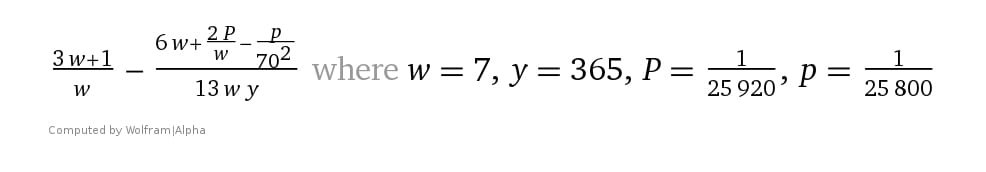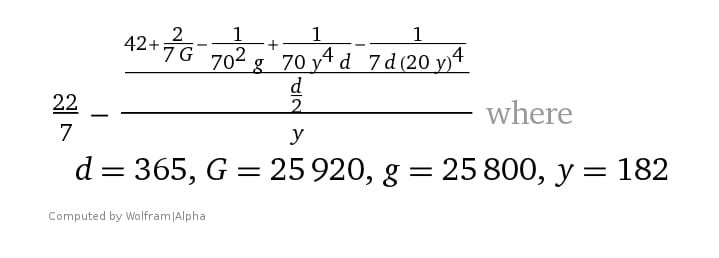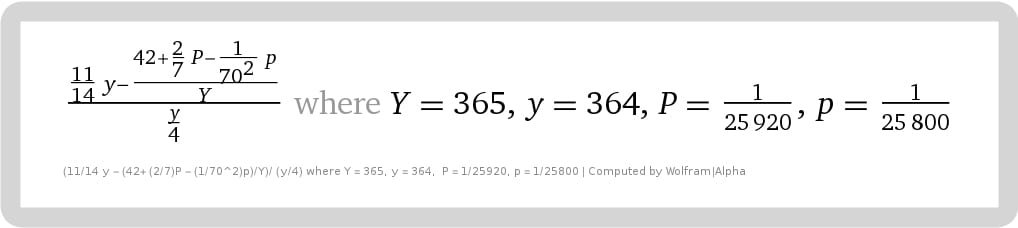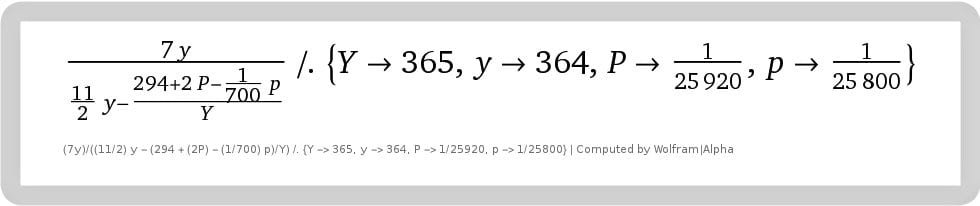# Rod of Pi π (Update)Using Enoch time frames, this formula is true to Pi to the 9th decimal of accuracy, (3.141 592 653 59).  The 22/7 is well known as an approximate of Pi, but here the 7/22 corresponds to the 7th day of the Creation and the 22nd day (i. e., the last day of Tabernacles).  This demonstrates the extreme compatibility of Pi with the Enochian calendar. (In the Bible a day is as a year and a year as a day.)Note the seven-sevens (“w“) when this same formula is written fully: www/w + 1/w – 6w/13wyPi of time, accurate to 18 decimals of pi. The last two terms represent the smallest division of a day according to ancient Jewish and Babylonian sources

The equation is arranged as “A time, times, and a half-time” (“d, G, g, y”), Dan 9:27, 12:7, Rev 12:14. The two largest numbers (“G” and “g”) refer to One Year of Precession, but also, reversely, to the smallest part of a day. The “182” is half an Enochian year of 364 days

##### The Rod of π (Pi)The Milky Way. The “1/25920 and 1/25800” in the formula refers to both the smallest Jewish/Babylonian parts of a year (= 3.333… seconds) and the largest, known as “The Great Year” of precession, (= 72 & 71.666… years = 1 day), that is, when “d” is redefined as “The Great Year * d”. Hence the formula tracks the sun, moon, and stars, measuring the width of the entire galaxy to an accuracy of about the width of a few hairs! It thus measures light-YEARS, too, because the formula refers to both time and space

Accurate to the diameter of π to the 25th decimal, the formula incorporates all biblical timeframes and is the frame upon which all events occur  — especially Biblical history.Accurate to Pi rounded to the 25th decimal  = 3.141 592 653 589 793 238 462 643 4 — ten billion times more accurate than what NASA uses for its space explorations. To verify, copy:  ((22 y)/7 – (42 + 2/(7 G) – 1/(70^2 g) + (1/(70*y^4 d)) – 1/(7d (20y)^4)) /(d/2))/y, where d -> 365, G -> 25920, g -> 25800, y -> 182 , and paste hereAccurate to Pi rounded to the 25th decimal  = 3.141 592 653 589 793 238 462 643 417 — ten billion times more accurate than what NASA uses for its space explorations. To verify, copy:  ((22 y)/7 – (42 + 2/(7 G) – 1/(70^2 g) + (1/(70*y^4 d)) – 1/(7d (20y)^4)) /(d/2))/y, where d -> 365, G -> 25920, g -> 25800, y -> 182 , and paste hereThis is an expression of the lowest common denominator of the massive fraction 163017430328214532876984603/51890059693747993536000000 that expresses the above (Pi to the 25th decimal). It  demonstrates the compatibility with the Enochian calendar even at the final step

The rest is explained here.

The following was written before the above formula (completed by Sept 8, 2019) had fully been worked out. It is still accurate, just not complete. If nothing else it shows the development in my thinking.

The below formula is true to Pi π by an astonishing 1/1,000,000,000,000,000,000

This is nearly 1000 times more accurate than what NASA uses for all its space endeavors. Yet it unfolds Enoch’s calendar (and all calendars) and is the numerical sum of much that is found in nature and the Bible.

As discussed in previous documents, (written before this formula was discovered)…

• 360 is the prophetic year (see 360calendar.com)
• 364 is a year on Enoch’s calendar
• 365 is the rounded solar Year (Egyptian Cal.)
• 25920 and 25800 years are the “Great Year“, that is, Precession of the Equinox. And as the 1/25920th part of a day (and thus 1/25800th, too), it is the smallest unit according to Babylonian and Rabinnic reckoning (= 3.33… seconds)Pi: The Rod of Enoch. This formula locates a position in space/time of a straight line surrounded by its circle, which by definition is π. This equals Cir 178087601141201/Dia 56,687,044,050,000

## The Logical Next Step Towards true Pi

Increases accuracy several decimals to about 7/1,000,000,000,000,000,000,000.

While the addition of “1/12^6t-P”  to the formula works well, it does not increase accuracy towards Pi as dramatically as the simpler formula.Accuracy to π is 6.6… × 10^-21. Elegantly, the “P” in “1/12^6*360–P” is itself 1/12*6*360 days or years. Or another comparison:  12^6*360 = 1.6*25920^2

The rod of God is One. Revelation 11:1-14 (and 12:6, 14) measures time as “1260 days”, or “42 months”, or 2/7 (“3.5 years”), or as “a time” (365), “times” (364 + 360), “and the dividing of time” (180 or apart thereof, i.,e 1/25920 or 1/25800). All this, in turn, is based upon Daniel’s 70 x 7, and Enoch’s 70 x 70. Compare the red to the formula.

`Main Formula, accurate to Pi to the 20th decimal ( = 3.141592653589793238456 — 10,000 times more accurate than what NASA uses for all its space explorations. To verify, copy:  (11/14y – (42 + 2/7P – 1/70^2p + 1/(12^6*t)P)/Y)/(y/4) where Y=365, y=364, t=360, P=1/25920, p=1/25800) , and paste here. The “y/4” at bottom of formula divides Enoch’s solar year into its four seasons (364/4=91 days)`

Therefore, this addition to the formula is an extremely flexible choice. It is, therefore, the logical further division of the smallest part of a day, (3.333… seconds; i.e., 1/25920th of a day). Moreover, “t = 360” can represent exactly one degree of the circle (π), as well as to units of measurement of time and space.

The final fraction for this formula is large:

Circumference 153148817550530282861117/diameter 48748782683691417600000

The importance of 360 in the diameter is obvious:= 48748782683691417600000. In the Bible, 3, 7, and 10 are considered perfect numbers. All Bible numbers go into this large number evenly, including 2300 when understood as 2295.5 (i.e., “evenings and mornings”, Dan 8) which follows the 10% rule from 7*365. Example: (4,200,000, 700,000, 1,950,000, 325,000, 20,000, 18,000 etc. -> total lengths/perimeters of future territory in cubits or rods in Ezekiel and ALL other proportions), 144,000, (48000-> perimeter of New Jerusalem in Book of Revelation), 16,000, (“A thousand generations”, as 100,000, or 70,000, or 40,000 years, Deut 7:9″), (both precessions 25920, 25800), 7000, 2295.5, 1290, 2555, 1274, 1260, 490, 430, 390, 360, 364, 365, 336, 70. 65, etc. The only exception appears to be 1335 of Daniel 12, although the difference between 1260 and 1335 is 75, the number that appears prominently in the above formula

Therefore, the various orbital lengths of the earth and moon in relation to the sun and other stars appear related to Pi, as well as the other planets in our solar system due to orbital resonance, with earth, apparently the fulcrum.

##### Derivatives of above formulaBasic formula: Here converted into units of seven (“weeks”) instead of “one” that reveal other relationships to the Enoch calendar. (Example, “the week” and “294” (7*7*6) are crucial cycles in the calendar. Moreover, keep in mind that a day is as a year (or “a 1000 years”) and a year is as a day”, (2Peter 3:8)
###### The formula multiplied by 1 season of Enoch (91)An expanded version of the basic formula for Enoch’s  Pi in space and time. Keep in mind that “1274” is simply 364*3.5, and is, therefore, the equivalent of 1260 days (Rev. 11). The 1000 years begins the last Sabbatical cycle of seven-year periods that “cuts short” 21 weeks, less “the twinkling of an eye” of the full 1001 years. In part, it has to do with the tithe (10%). Example, 441 + 49 = 490 (Dan 9); 7*365 less 10% = 2300 (Dan 5,8,12), and here, +/- 10% of 1000 (and 1oo1) x 365 (0r 360 or 364) (&*4), etc.

### The continual fractions best display the connection of Pi to the Rod of Enoch (and his calendar)

I will elaborate later on how the rationalization of π using continual fractions unravels the above formula in stunning stages.

Example, take the sixth fraction of 104348/33215. This means that the perimeter is 33215*4, which is 364*365. This is just one example.

Fractions: Approximate fractions include (in order of increasing accuracy)

22/7,

333/106,

355/113,

52163/16604,

103993/33102,

104348/33215,

and… 245850922/78256779.  Wiki

Click here for the signs on earth and in the heavens in regard to the revealing of this formula: “Hurricane Dorian, the Eye of Pi & Sun/Moon”Hurricane hunter plane in the eye of Dorian with the sun in view within the circle of the eye. Amazingly, at the same time, on the sun (too bright to see) is a large number 7 within the Pi π symbol literally sketched on the sun!

## One thought on “Rod of Pi π (Update)”

1.Pi says:

Help!